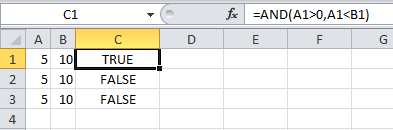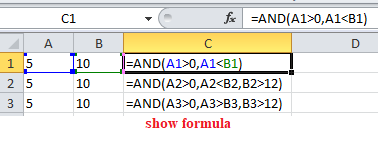How to use Excel AND Function

This Excel tutorial explains how to use the AND function with syntax and examples.

Excel AND Function Description

Microsoft Excel AND function tests a number of supplied conditions and returns TRUE if all conditions are TRUE. It returns FALSE if any of the conditions are FALSE.

That is;

• TRUE if ALL of the conditions evaluate to TRUE
Also See:   Extract data with helper column in Excel

or

• FALSE otherwise (i.e. if ANY of the conditions evaluate to FALSE).

Excel And Function Examples

The following spreadsheet shows three examples of the Excel And function:Explanation:  Based on the example above, the AND function returns;

• the function in cell C1 evaluates to TRUE, as BOTH of the supplied conditions are TRUE;
• the function in cell C2 evaluates to FALSE, as the third condition, B2>12, is FALSE;
• the function in cell C3 evaluates to FALSE, as ALL of the supplied conditions are FALSE.
Also See:   Highlight row and column intersection exact match in Excel

Syntax

AND( logical_test1, [logical_test2], … )

Arguments

where the logical_test arguments are one or more conditions that evaluate to either TRUE or FALSE.

Notes:

• In current versions of Excel (Excel 2007 and later), you can enter up to 255 logical_test arguments to the Excel And function. However, in Excel 2003, the function can only handle up to 30 arguments.
• If the logical_test arguments evaluate to numbers, instead of logical values, the value zero is treated as FALSE and all non-zero values are treated as TRUE.